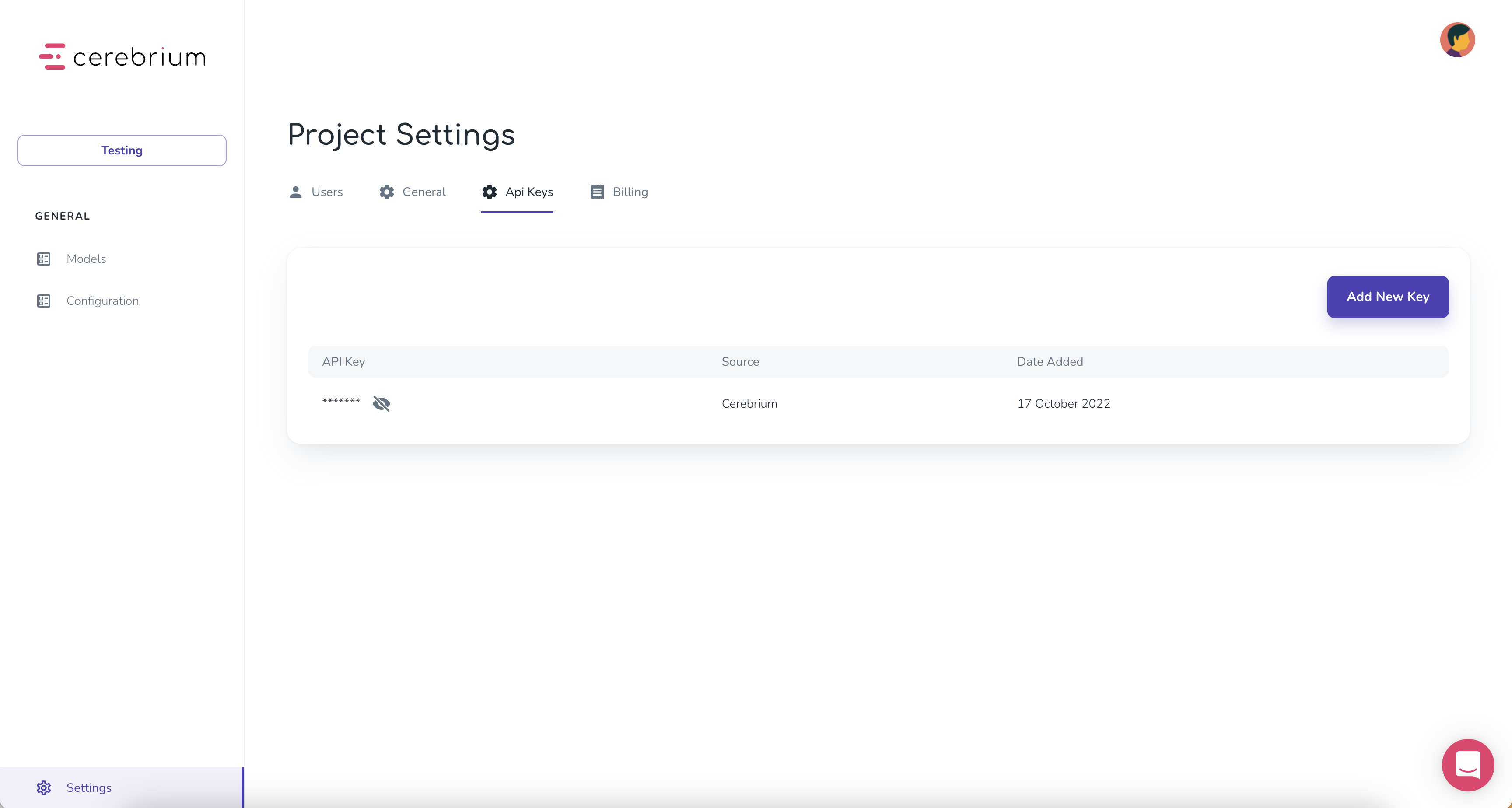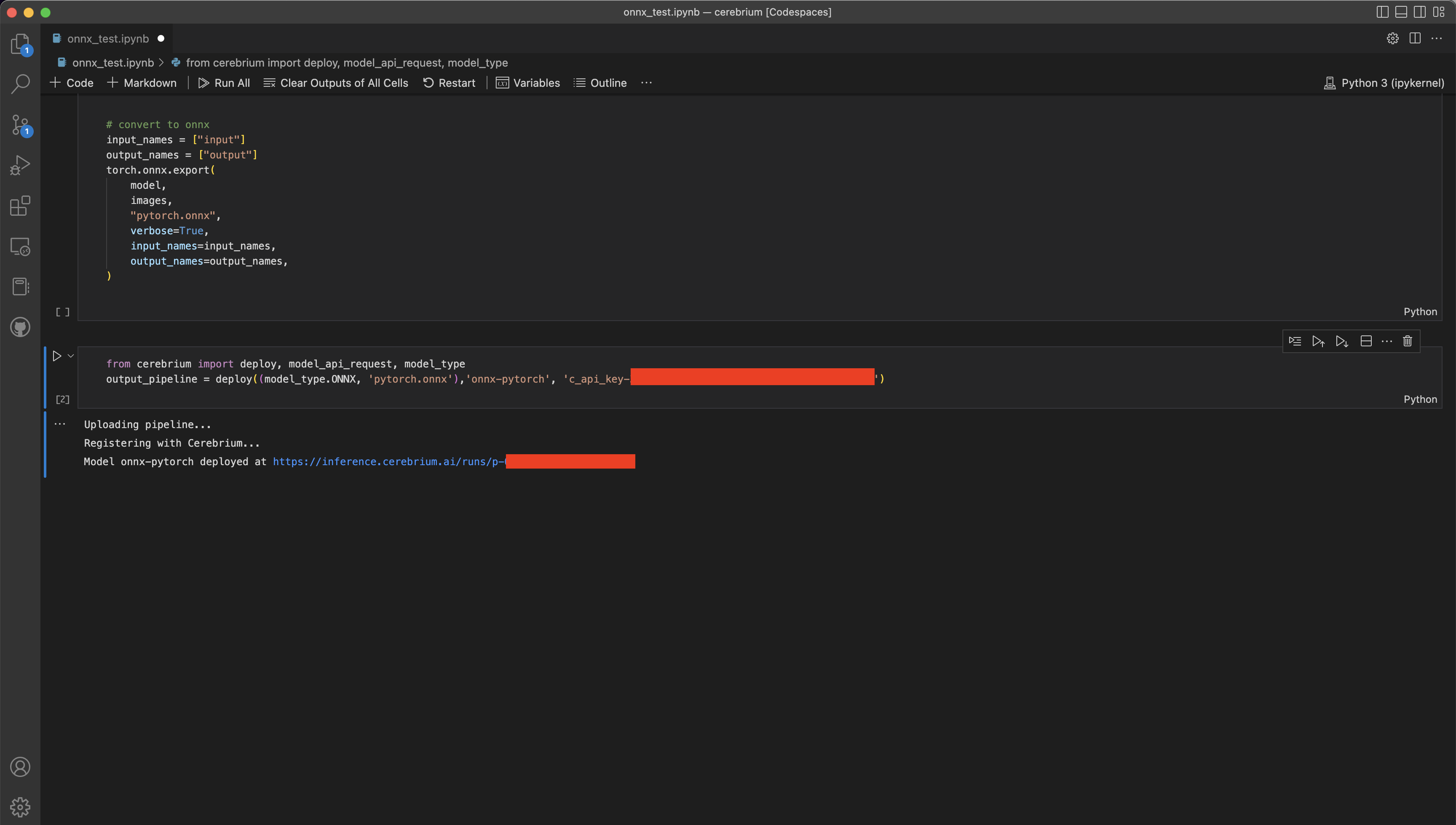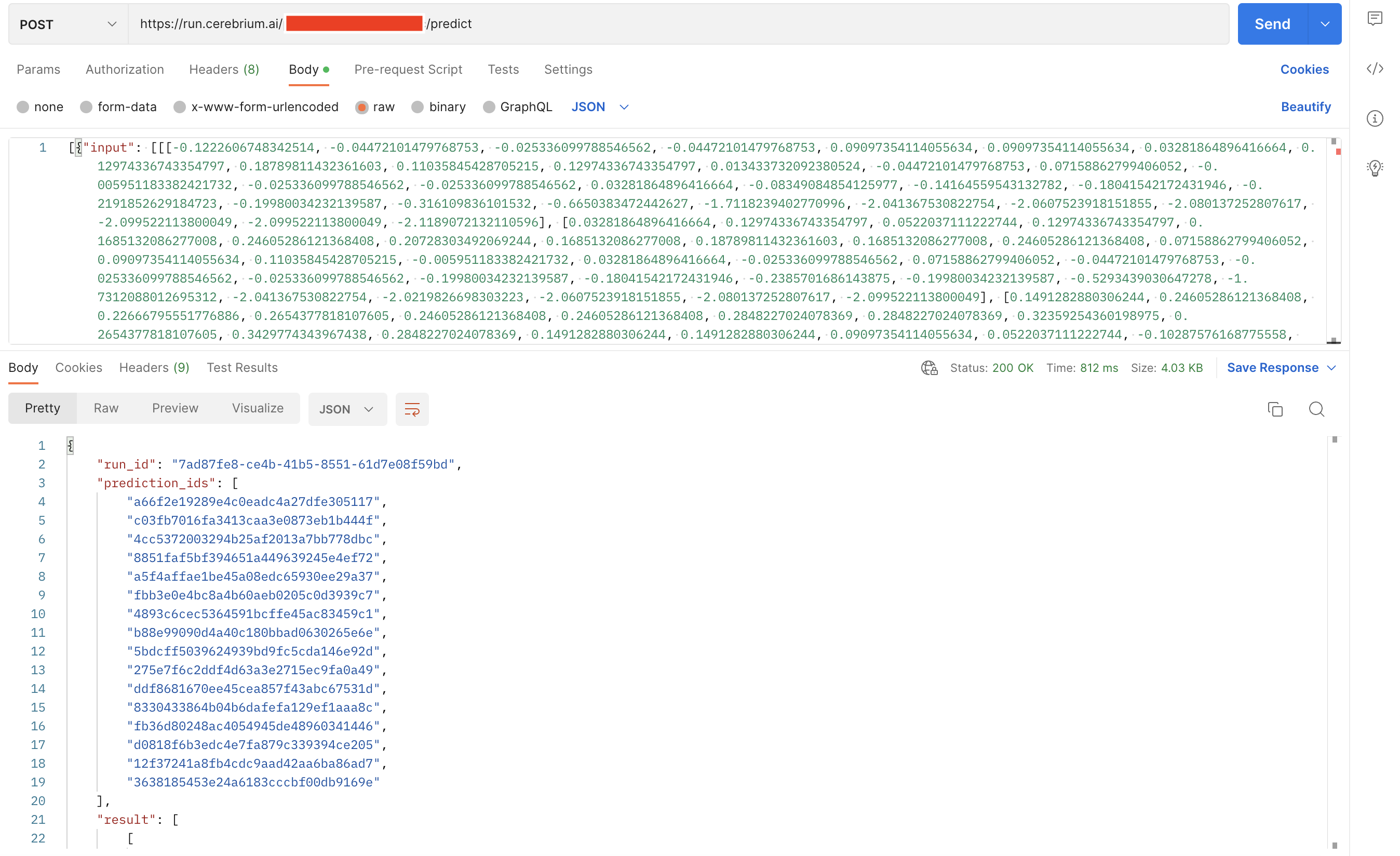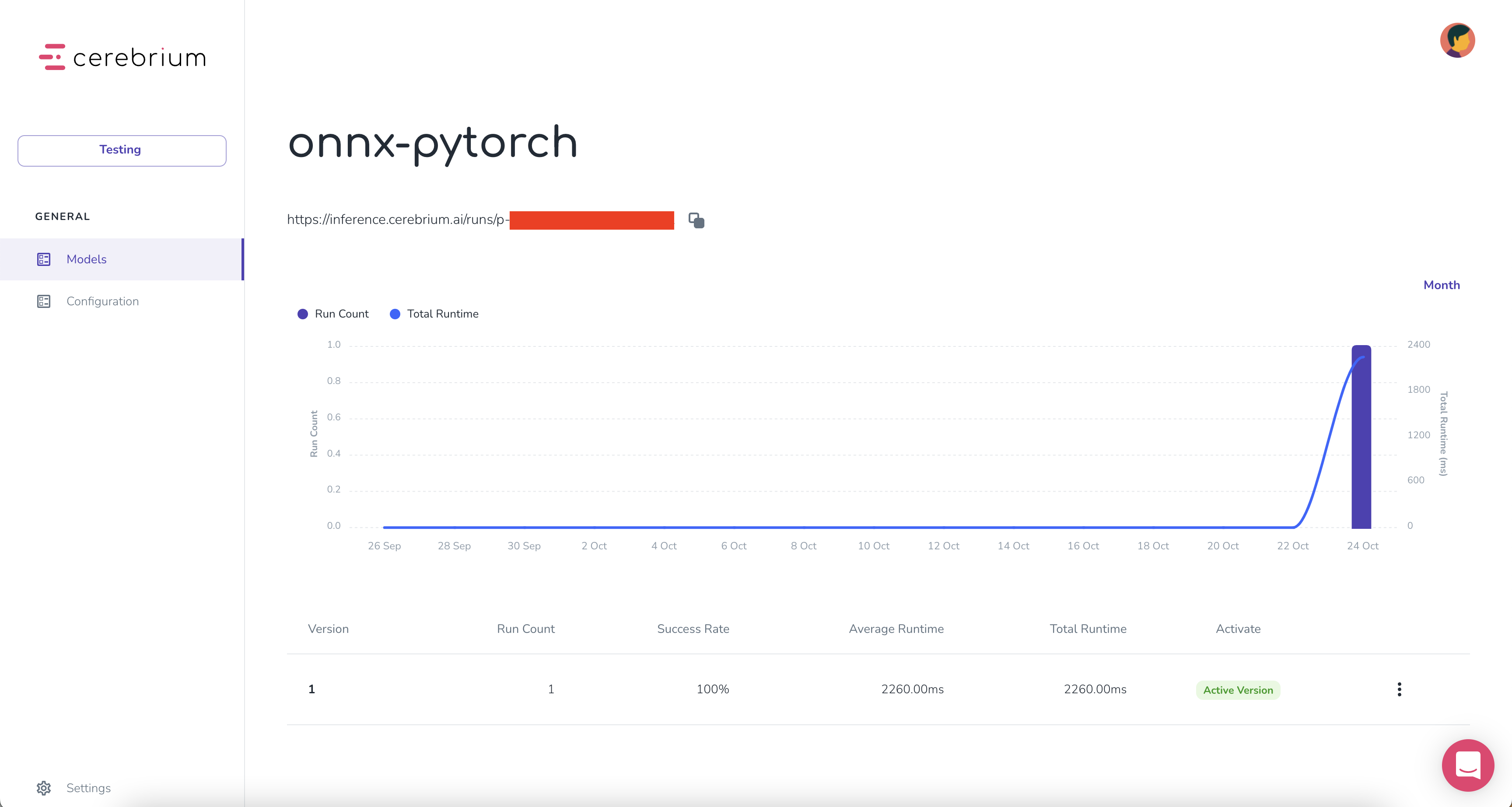## ​Intro

ONNX is an open format built to represent machine learning models. ONNX defines a common set of operators - the building blocks of machine learning and deep learning models - and a common file format to enable AI developers to use models with a variety of frameworks, tools, runtimes, and compilers.

By the end of this guide you’ll have an API endpoint that can handle any scale of traffic by running inference on serverless CPU’s/GPU’s.

### ​Project set up

Before building you need to set up a Cerebrium account. This is as simple as starting a new Project in Cerebrium and copying the API key. This will be used to authenticate all calls for this project.

### ​Create a project

1. Go to dashboard.cerebrium.ai
3. Navigate to the API Keys page
4. You should see an API key with the source “Cerebrium”. Click the eye icon to display it. It will be in the format: c_api_key-xxx### ​Develop model

Now navigate to where your model code is stored. This could be in a notebook or in a plain `.py` file.

To start you should install the Cerebrium framework running the following command in your notebook or terminal. You will need the optional dependency `onnxruntime` to run the model locally.

``````pip install cerebrium[onnxruntime]
``````

If you are on a GPU machine you can also install the GPU version of the runtime instead.

``````pip install cerebrium[onnxruntime-gpu]
``````

Copy and paste our code below. This creates a simple Convolutional Neural Network. This code could be replaced by any Pytorch model. Make sure you have the required libraries installed.

``````import torch
import torch.nn as nn
import torchvision
import torchvision.transforms as transforms

batch_size = 64
num_classes = 10
learning_rate = 0.001
num_epochs = 2

device = torch.device('cuda' if torch.cuda.is_available() else 'cpu')

# Use transforms.compose method to reformat images for modeling,
# and save to variable all_transforms for later use
all_transforms = transforms.Compose([transforms.Resize((32,32)),
transforms.ToTensor(),
transforms.Normalize(mean=[0.4914, 0.4822, 0.4465],
std=[0.2023, 0.1994, 0.2010])
])
# Create Training dataset
train_dataset = torchvision.datasets.CIFAR10(root = './data',
train = True,
transform = all_transforms,

# Create Testing dataset
test_dataset = torchvision.datasets.CIFAR10(root = './data',
train = False,
transform = all_transforms,

# Instantiate loader objects to facilitate processing
batch_size = batch_size,
shuffle = True)

batch_size = batch_size,
shuffle = True)

# Create Neural Network
class ConvNeuralNet(nn.Module):
def __init__(self, num_classes):
super(ConvNeuralNet, self).__init__()
self.conv_layer1 = nn.Conv2d(in_channels=3, out_channels=32, kernel_size=3)
self.conv_layer2 = nn.Conv2d(in_channels=32, out_channels=32, kernel_size=3)
self.max_pool1 = nn.MaxPool2d(kernel_size = 2, stride = 2)

self.conv_layer3 = nn.Conv2d(in_channels=32, out_channels=64, kernel_size=3)
self.conv_layer4 = nn.Conv2d(in_channels=64, out_channels=64, kernel_size=3)
self.max_pool2 = nn.MaxPool2d(kernel_size = 2, stride = 2)

self.fc1 = nn.Linear(1600, 128)
self.relu1 = nn.ReLU()
self.fc2 = nn.Linear(128, num_classes)

def forward(self, x):
out = self.conv_layer1(x)
out = self.conv_layer2(out)
out = self.max_pool1(out)

out = self.conv_layer3(out)
out = self.conv_layer4(out)
out = self.max_pool2(out)

out = out.reshape(out.size(0), -1)

out = self.fc1(out)
out = self.relu1(out)
out = self.fc2(out)
return out

model = ConvNeuralNet(num_classes)

# Create loss function and optimizer
criterion = nn.CrossEntropyLoss()

optimizer = torch.optim.SGD(model.parameters(), lr=learning_rate, weight_decay = 0.005, momentum = 0.9)

# Train our model
for epoch in range(num_epochs):
for i, (images, labels) in enumerate(train_loader):
images = images.to(device)
labels = labels.to(device)

outputs = model(images)
loss = criterion(outputs, labels)

loss.backward()
optimizer.step()

print('Epoch [{}/{}], Loss: {:.4f}'.format(epoch+1, num_epochs, loss.item()))

correct = 0
total = 0
images = images.to(device)
labels = labels.to(device)
outputs = model(images)
_, predicted = torch.max(outputs.data, 1)
total += labels.size(0)
correct += (predicted == labels).sum().item()

print('Accuracy of the network on the {} train images: {} %'.format(50000, 100 * correct / total))

# convert to onnx
input_names = ["input"]
output_names = ["output"]
torch.onnx.export(
model,
images,
"pytorch.onnx",
verbose=True,
input_names=input_names,
output_names=output_names,
)
``````

In the last line of code, you will see we exported the Pytorch model to an Onnx format. This is all you need to deploy your model to Cerebrium! You can then import the `deploy()` function from the Cerebrium framework. I used the CloudPickle function to save my model below.

``````from cerebrium import deploy, model_type
output_flow = deploy((model_type.ONNX, "pytorch.onnx"),"onnx-pytorch", "<API_KEY>")
``````Your model is now deployed and ready for inference all in under 10 seconds! Navigate to the dashboard and on the Models page you will see your model.

You can run inference using `curl`

``````curl --location --request POST '<ENDPOINT>' \